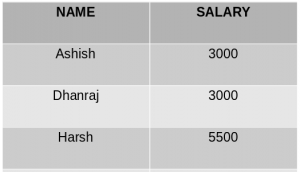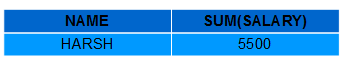# SQL | GROUP BY

The GROUP BY Statement in SQL is used to arrange identical data into groups with the help of some functions. i.e if a particular column has same values in different rows then it will arrange these rows in a group.

Important Points:

• GROUP BY clause is used with the SELECT statement.
• In the query, GROUP BY clause is placed after the WHERE clause.
• In the query, GROUP BY clause is placed before ORDER BY clause if used any.

Syntax:

```SELECT column1, function_name(column2)
FROM table_name
WHERE condition
GROUP BY column1, column2
ORDER BY column1, column2;

function_name: Name of the function used for example, SUM() , AVG().
table_name: Name of the table.
condition: Condition used.
```

Sample Table:

EmployeeStudent

• Group By single column: Group By single column means, to place all the rows with same value of only that particular column in one group. Consider the query as shown below:
```SELECT NAME, SUM(SALARY) FROM Employee
GROUP BY NAME;
```

The above query will produce the below output:As you can see in the above output, the rows with duplicate NAMEs are grouped under same NAME and their corresponding SALARY is the sum of the SALARY of duplicate rows. The SUM() function of SQL is used here to calculate the sum.

• Group By multiple columns: Group by multiple column is say for example, GROUP BY column1, column2. This means to place all the rows with same values of both the columns column1 and column2 in one group. Consider the below query:
```SELECT SUBJECT, YEAR, Count(*)
FROM Student
GROUP BY SUBJECT, YEAR;
```

Output:As you can see in the above output the students with both same SUBJECT and YEAR are placed in same group. And those whose only SUBJECT is same but not YEAR belongs to different groups. So here we have grouped the table according to two columns or more than one column.

HAVING Clause

We know that WHERE clause is used to place conditions on columns but what if we want to place conditions on groups?

This is where HAVING clause comes into use. We can use HAVING clause to place conditions to decide which group will be the part of final result-set. Also we can not use the aggregate functions like SUM(), COUNT() etc. with WHERE clause. So we have to use HAVING clause if we want to use any of these functions in the conditions.

Syntax:

```SELECT column1, function_name(column2)
FROM table_name
WHERE condition
GROUP BY column1, column2
HAVING condition
ORDER BY column1, column2;

function_name: Name of the function used for example, SUM() , AVG().
table_name: Name of the table.
condition: Condition used.
```

Example:

```SELECT NAME, SUM(SALARY) FROM Employee
GROUP BY NAME
HAVING SUM(SALARY)>3000;
```

Output:As you can see in the above output only one group out of the three groups appears in the result-set as it is the only group where sum of SALARY is greater than 3000. So we have used HAVING clause here to place this condition as the condition is required to be placed on groups not columns.

This article is contributed by Harsh Agarwal. If you like GeeksforGeeks and would like to contribute, you can also write an article using contribute.geeksforgeeks.org or mail your article to contribute@geeksforgeeks.org. See your article appearing on the GeeksforGeeks main page and help other Geeks.